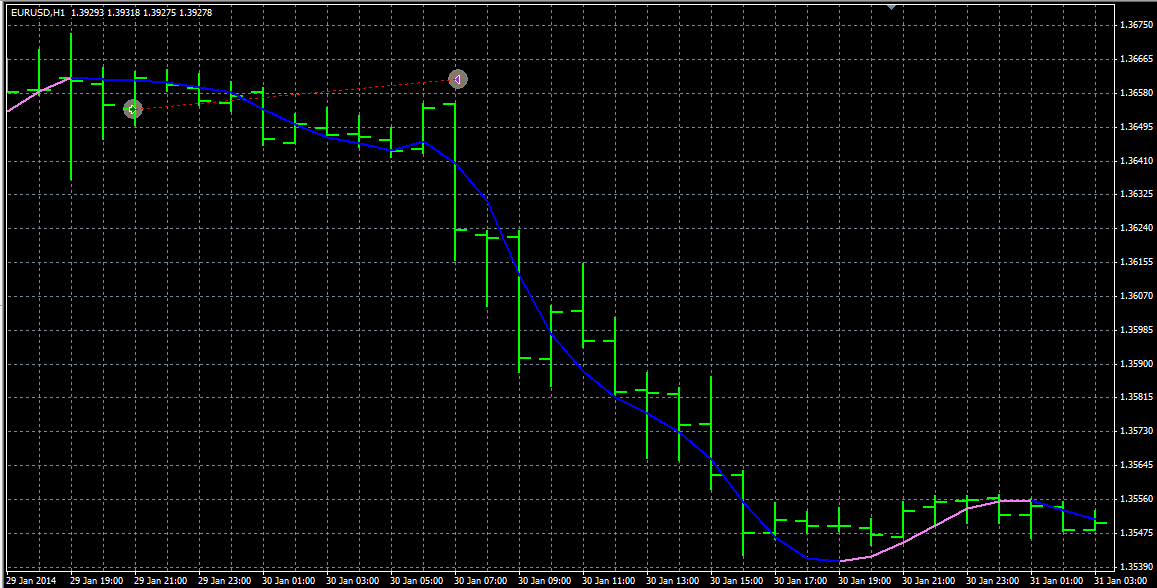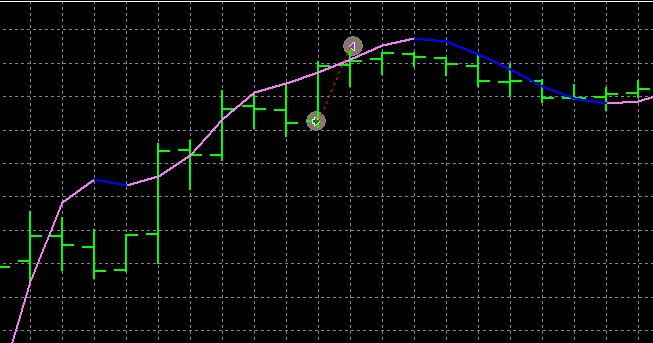# Slope Direction Line problem38

Hi all,

I was trying a simple close condition when the Slope Direction Line changes from going downwards to going upwards.

In some cases, it does not work, and I really do not understand why.

I get the value of the Slope (shifted by 1 to have a consolidated value from the last bar), and compare it to the already stored value from the iteration before.

The prints say values are correct, but this is definitely what I expect (see image).

Parts of the code:

double slopeSLOW_Plus = iCustom (Symbol(), PERIOD_CURRENT, "Slope_Direction_Line", SlopeSLOW_Period, SlopeSLOW_Method, SlopeSLOW_Price, 0, 1);

double slopeSLOW_Minus = iCustom (Symbol(), PERIOD_CURRENT, "Slope_Direction_Line", SlopeSLOW_Period, SlopeSLOW_Method, SlopeSLOW_Price, 1, 1);

double slopeFAST_Plus = iCustom (Symbol(), PERIOD_CURRENT, "Slope_Direction_Line", SlopeFAST_Period, SlopeFAST_Method, SlopeFAST_Price, 0, 1);

double slopeFAST_Minus = iCustom (Symbol(), PERIOD_CURRENT, "Slope_Direction_Line", SlopeFAST_Period, SlopeFAST_Method, SlopeFAST_Price, 1, 1);

...

if ( (slopeFAST_Minus_OLD!=EMPTY_VALUE) && (slopeFAST_Plus!=EMPTY_VALUE) )

{

}

Results are often awkward: what could it be?(in one case it also opened the trade (sell) while the slope was going up, and I see NO slope change!!!)Files:
05.png 21 kb
04.png 7 kb197182

Hi all,

I was trying a simple close condition when the Slope Direction Line changes from going downwards to going upwards.

In some cases, it does not work, and I really do not understand why.

I get the value of the Slope (shifted by 1 to have a consolidated value from the last bar), and compare it to the already stored value from the iteration before.

The prints say values are correct, but this is definitely what I expect (see image).

Parts of the code:

Results are often awkward: what could it be?(in one case it also opened the trade (sell) while the slope was going up, and I see NO slope change!!!)Try something like this :

double slope_p3 = iCustom (Symbol(), PERIOD_CURRENT, "Slope_Direction_Line", SlopeSLOW_Period, SlopeSLOW_Method, SlopeSLOW_Price, 0, 3);

double slope_p2 = iCustom (Symbol(), PERIOD_CURRENT, "Slope_Direction_Line", SlopeSLOW_Period, SlopeSLOW_Method, SlopeSLOW_Price, 0, 2);

double slope_p1 = iCustom (Symbol(), PERIOD_CURRENT, "Slope_Direction_Line", SlopeSLOW_Period, SlopeSLOW_Method, SlopeSLOW_Price, 0, 1);

double diffp = slope_p2-slope_p3;

double diffc = slope_p1-slope_p2;

if (diffp*diffc<0)

{

if (diffc>0) // code fore buy

if (diffc<0) // code fore sell

}38

Try something like this :
double slope_p3 = iCustom (Symbol(), PERIOD_CURRENT, "Slope_Direction_Line", SlopeSLOW_Period, SlopeSLOW_Method, SlopeSLOW_Price, 0, 3);

double slope_p2 = iCustom (Symbol(), PERIOD_CURRENT, "Slope_Direction_Line", SlopeSLOW_Period, SlopeSLOW_Method, SlopeSLOW_Price, 0, 2);

double slope_p1 = iCustom (Symbol(), PERIOD_CURRENT, "Slope_Direction_Line", SlopeSLOW_Period, SlopeSLOW_Method, SlopeSLOW_Price, 0, 1);

double diffp = slope_p2-slope_p3;

double diffc = slope_p1-slope_p2;

if (diffp*diffc<0)

{

if (diffc>0) // code fore buy

if (diffc<0) // code fore sell

}

I got the rationale, but I think it needs a bit of workaround:

when slope is in the opposite direction as the one we are checking, the buffer is filled with EMPTY_VALUE (which might be machine dependent, not sure, in my case is 2147483647).

So, the most straightforward thing I see is that "slope_p2-slope_p3" will always be 0

The other is absurd values when it changes slope (EMPTY_VALUE - reasonable value).

Just tested, to be sue I was't telling BS.

I will think about itmaybe a tweak of the indicator will do, not sure...

Ah, another thing: do you thing is thus better to check 3 bars, not just 2?197182

I got the rationale, but I think it needs a bit of workaround:

when slope is in the opposite direction as the one we are checking, the buffer is filled with EMPTY_VALUE (which might be machine dependent, not sure, in my case is 2147483647).

So, the most straightforward thing I see is that "slope_p2-slope_p3" will always be 0

The other is absurd values when it changes slope (EMPTY_VALUE - reasonable value).

Just tested, to be sue I was't telling BS.

I will think about itmaybe a tweak of the indicator will do, not sure...

Ah, another thing: do you thing is thus better to check 3 bars, not just 2?

That code is checking 3 bars not 2 (it is checking if the slope has changed, and for that it needs to use values of 3 bars)

As of values : I am not familiar with the indicator you use. Usually slope direction line is a simple hull moving average but with some repainting, so it might be a good idea to use a correctly coded hull averages instead. But if the indicator that you use has a correct value in the first buffer, that code snippet must work OK38

The indicator I was referring to is named exactly "Slope direction Line" and is published in many places around the web, don't remember where I initially got it from but I found it back here: https://www.mql5.com/en/code/7751

It has two buffers, one for the uptrend and one for the downtrend: it only fills the one according to the direction, the other is EMPTY_VALUE.

It is a slight variation of the Hull MA indeed, at least in painting, because I am realising that it behaves (almost?) the same even if the colour does not change on the graph.

I could switch to Hull MA if I found something compatible...

I saw many threads here, I attached the "Hull Trend" here seems to draw what I need, but I am confused on which buffer to check to detect the slope change:

https://www.mql5.com/en/forum/172972

I then took the indicator you posted here: https://www.mql5.com/en/forum/173260/page2

Can I use with this indicator a snippet similar to the one in the post above?197182

The indicator I was referring to is named exactly "Slope direction Line" and is published in many places around the web, don't remember where I initially got it from but I found it back here: https://www.mql5.com/en/code/7751

It has two buffers, one for the uptrend and one for the downtrend: it only fills the one according to the direction, the other is EMPTY_VALUE.

It is a slight variation of the Hull MA indeed, at least in painting, because I am realising that it behaves (almost?) the same even if the colour does not change on the graph.

I could switch to Hull MA if I found something compatible...

I saw many threads here, I attached the "Hull Trend" here seems to draw what I need, but I am confused on which buffer to check to detect the slope change:

https://www.mql5.com/en/forum/172972

I then took the indicator you posted here: https://www.mql5.com/en/forum/173260/page2

Can I use with this indicator a snippet similar to the one in the post above?

Yep, that is the "slope direction line" that is actually hull moving average (and that version of slope direction line can repaint)

As of hull : simplest is to use the one from this post https://www.mql5.com/en/forum/174961/page13 and check the trend buffer (buffer index 3). When it is 1, the trend is up, when it is -1 the trend is down, when the current is not equal to previous the trend is changing38